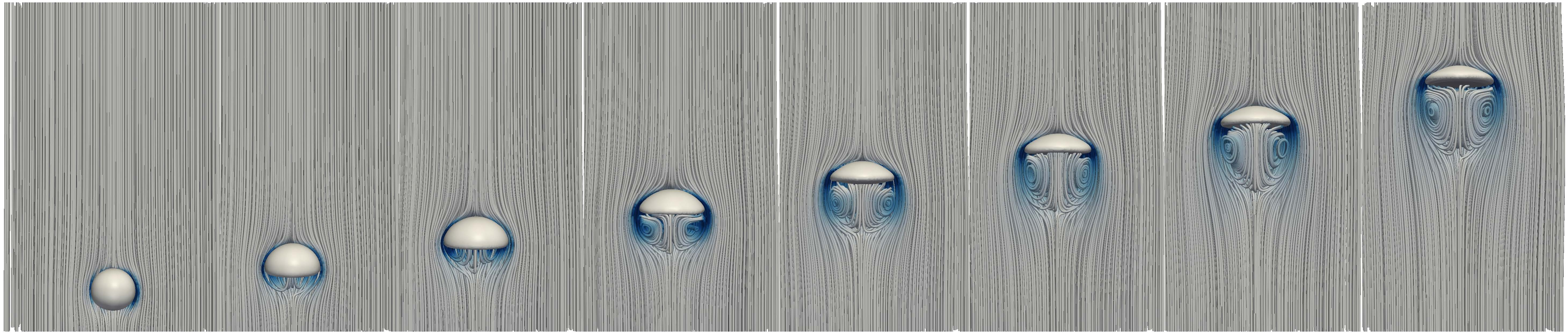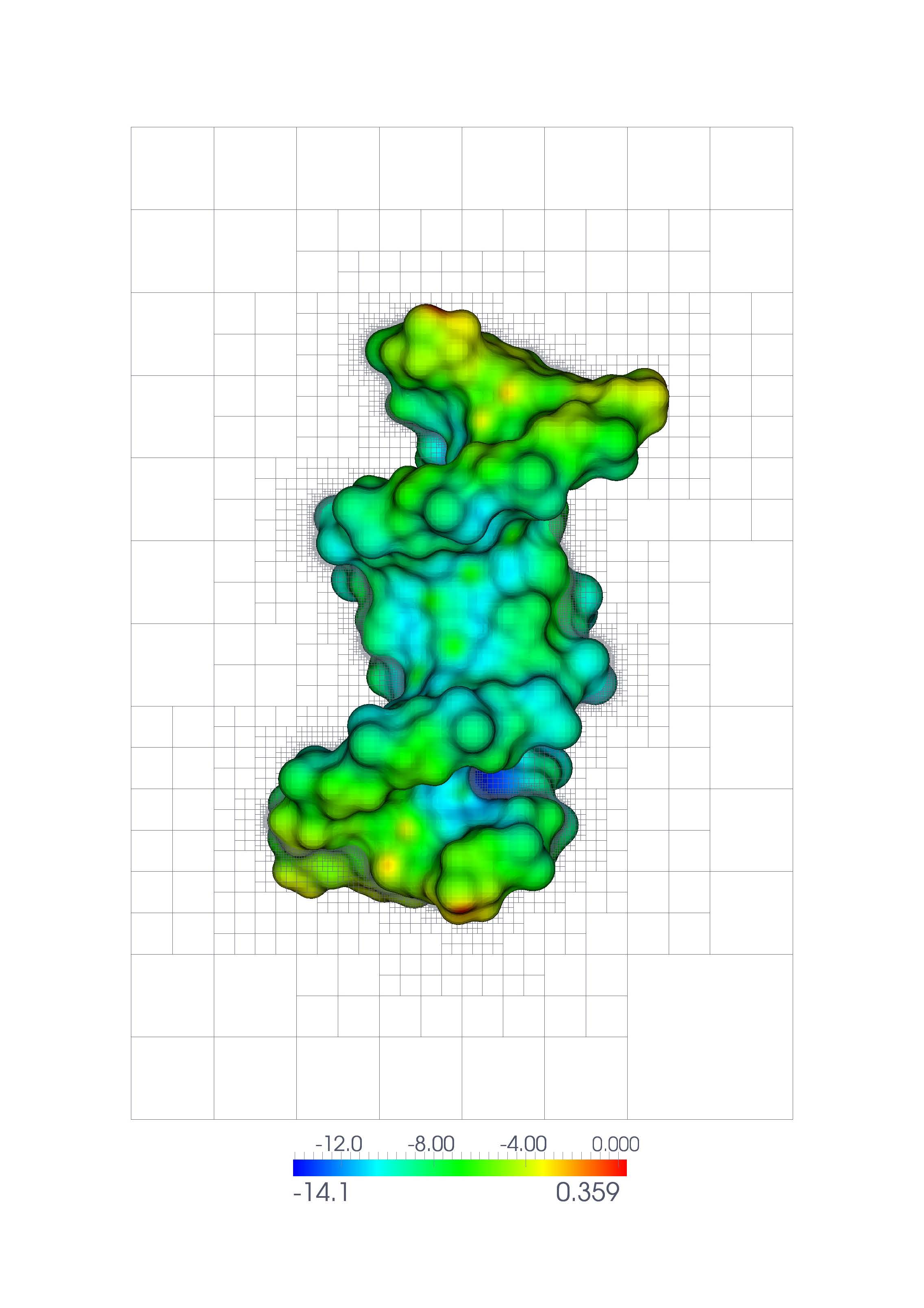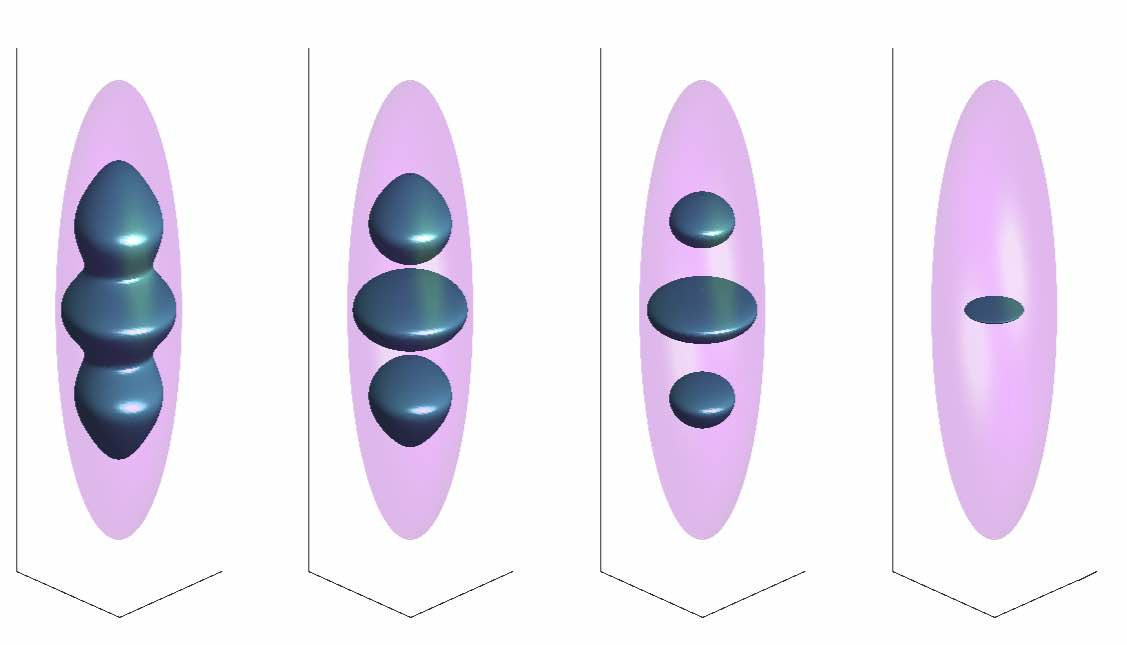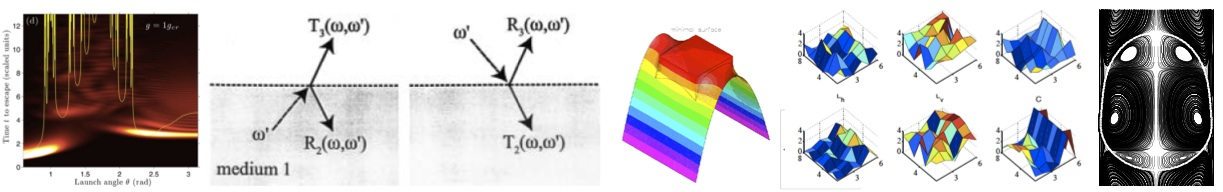# ResearchApplied mathematical science involves the use of analytical and computational mathematics to solve real-world problems. Its core is comprised of modeling, analysis and scientific computing. Using that core, applied mathematical scientists study a broad spectrum of problems across several disciplines.

In fact, applied mathematicians are connected more closely through their shared approach and attitude toward interdisciplinary research rather than a shared interest in any particular set of problems. Moreover, an explicit goal of applied mathematical science is to contribute significantly to another discipline. Hence, the objective of applied mathematics is to foster interdisciplinary research and education.

Research and education in applied mathematical science involves four stages:

• Find an interesting problem that may benefit from mathematical analysis.
• Develop an abstract model (i.e., a "mathematical model") that describes salient features of the problem.
• Apply existing analytical and computational methods or developing new methods to solve the mathematical model.
• Determine what insight the mathematical model has provided to the original problem.

Applied mathematicians are inherently interdisciplinary. As mentioned above, applied mathematicians are connected closely through their shared approach toward interdisciplinary research. They are individuals who are well trained in the development of mathematical models for real-world problems, and the modern analytical and computational tools to solve them. A strong group of applied mathematicians can be a great asset to any number of scientific and engineering programs within the university where they can provide the theoretical/quantitative support or foundation.Applied mathematics research requires a deep understanding of mathematics and broad knowledge of other disciplines. Applied mathematicians must be well-trained in fundamentals of mathematics to model, analyze and compute solutions to real-world problems.

Real-world mathematics problems usually are not amenable to complete abstraction. Therefore, they often do not fall within the admissible set of problems for "pure" mathematics research. Instead, applied mathematicians must work through these issues to respect the connections that any mathematical analysis will have to the application.

Because mathematics serves as the framework for so many different areas in science and engineering, applied mathematicians are continually increasing their breadth of knowledge in other fields.

In fact, applied mathematics research is usually assessed through two criteria: (1) the sophistication of the mathematics used and (2) the novelty of the application. At UC Merced, the faculty and students engage in research in the following areas:

 Mathematical Areas Application Areas Asymptotic Analysis and Perturbation Methods Dynamical Systems Numerical Analysis Free Boundary Problems Inverse Problems Nonlinear Analysis Nonlinear Waves Numerical Linear Algebra Numerical Optimization Stochastic and Statistical Modeling Waves in Random Media Acoustics BioelectricsBose-Einstein Condensates Computational Biology Economics Fluid Dynamics Image Processing and Compressed Sensing Mathematical Physics Plasma Physics Solar Science Ultrafast Laser and Nonlinear Optics Wireless Networks Dynamics and Design Problems for Circuits and Electromagnetic Media Weather Rrediction and Climate Modeling Computer Graphics